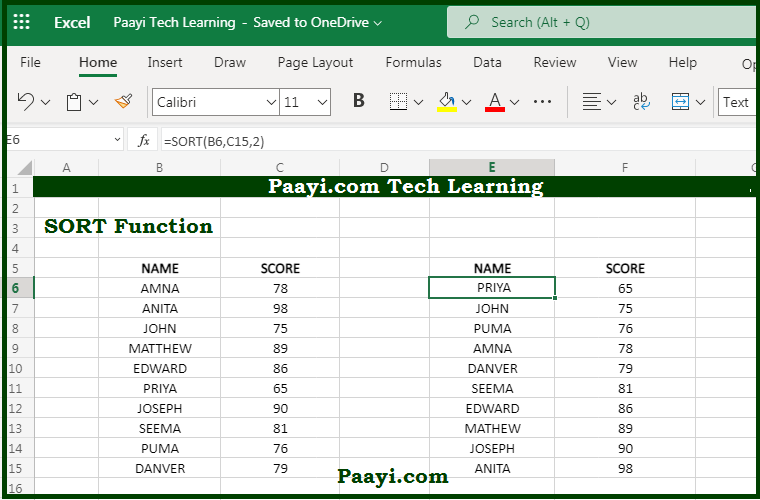# Learn How to Use Microsoft Excel SORT Function

Written by | 0 Comments | 651 Views

In this article, you will learn how to use the Microsoft Excel SORT function and its prime function in Microsoft Excel. You will also get to know the Microsoft Excel SORT function return value and syntax with the help of some examples.

Microsoft Excel SORT Function

The primary function of the Microsoft Excel SORT function is to sort a range or an array. That implies with the help of the SORT function; you can able to sort the content or range or an array. You can sort the values by one or more columns, and the SORT function returns the dynamic arrays of results.

Return Value of SORT Function

The return value will be sorted array.

Syntax of SORT Function

=SORT(array, [sort-index], [sort-order], [by-col])

Where the arguments:

array: This is the range or array you want to sort.

sort-index: This is the column index used for sorting, default is 1 (optional).

sort-order: 1 = Ascending, -1 = Descending, default is ascending order (optional).

by-col: TRUE = Sort by column, FALSE = sort by row, DEFAULT is FALSE (optional).

## How to Use Microsoft Excel SORT Function?With the Microsoft Excel SORT function, you can able to extract and sort the list of unique values from the range, and the result will be the dynamic arrays of values. In case the array is the final result, that means not handed off to another function. The array values will spill into a range that updates automatically when new unique values are added or subtracted from the source range or if the source value change.